# What is the Difference Between APR and APY In Crypto?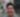Posted by: Kevin GrovesUpdated Jun 22nd, 2023

Key Takeaways:

• Annual Percentage Rate (APR) and Annual Percentage Yield (APY) are terms used to describe the amount of interest obtained on a crypto investment.
• APY differs from APR in that the latter does not take into account compounding interest.
• APR is generally better for borrowers, whilst APY is better for savers and investors who want a return.

The decentralized finance world often mentions APR and APY with staking and crypto bank accounts. But what do these terms actually mean? How are they calculated? Is it risky? This guide will explore the difference between APR and APY in crypto and how to understand them best.

## Overview of APR and APY In Crypto

It’s easy to get lost in all the terminology that comes with earning interest on Ethereum, stablecoins and many others. When investors put their hard-earned cryptocurrency into earning hubs, they lend their coins to someone else. This might be a broker or exchange (such as Coinbase or Binance), or directly to the blockchain network (like staking on the Solana protocol).

The easiest way to contextualize Annual Percentage Rate (APR) and Annual Percentage Yield (APY) in crypto is to think of them as a form of interest. Interest is like a fee for borrowing money. It is usually paid out as a percentage of the loan. For example, 3.5% on a 10k savings account would be \$10,350 by the end of the term if we looked exclusively at interest.

## Difference Between APR And APY In Crypto

APR and APY are terms given to calculations for determining the amount of interest on crypto investment. There are subtle differences in how the interest rate is calculated between the two, most notably the compounding frequency is not included in APR interest calculations. This means interest rates expressed as an APY are generally more lucrative than APR. Moreover, the interest APY advertised on some platforms does not factor in fees, compared to APY. Within the crypto industry, the term APY is more commonly used for loans, whereas APY is frequently used for interest savings accounts. The differences between APY and APY are summarized in the table below.

APRAPY
DefinitionAn APR is the same as an interest rate, except it typically accounts for any fees the institution charges.An APY accounts for the base interest rate, as well as compounding interest.
Includes compoudingNoYes
Includes feesMost of the timeNo

As an example, an APR of 10% that compounded weekly would actually be equivalent to an APY of 10.51%. It is worth noting that fees aren’t typically as relevant for Bitcoin and other crypto-earning products. APRs that calculate fees are aimed toward borrowers, not lenders.

Interest Type10.51% APY on BTC10% APR on BTC
Initial investment\$10,000 of BTC\$10,000 of BTC
Interest pay frequencyWeeklyAnnually
Balance after one year\$11,050.65 of BTC\$11,000 of BTC

The major difference between APR and APY is the compounding frequency which is a significant element of how much interest an earning opportunity will return. This is the number of times per year that interest is paid to the account. The frequency can be any amount of time, but it is usually divided into logical periods:

• Daily (365 times)
• Weekly (52 times)
• Fortnightly (26 times)
• Monthly (12 times)
• Quarterly (4 times)
• Bi-annually (2 times)
• Annually (1 time)

APR savings accounts do not account for compounding frequency in their listed rates. Some may not compound at all. The compounding frequency of a crypto savings account or staking coin will affect how much interest an investor can earn. The more frequent the compound interest rate is, the greater the potential yield and interest paid overall.

## Is APR or APY Better In Crypto?

There is no real ‘better’ when comparing APR and APY. Each method of calculating interest is suited to a specific consumer. APR is better for borrowers. When borrowing digital currencies like Bitcoin, some platforms will charge upfront fees on top of an interest rate. The quoted APR typically accounts for these fees and gives debtors a more accurate picture of what they will owe.

On the other hand, an APY calculation is better for lenders that want to earn compound interest. Investors that wish to engage in earning protocols like Nexo, Crypto.com, or Binance Earn will prefer an APY. This term includes compound interest and can be used to precisely predict potential profit.

## How Does Compound Interest In Crypto Work?

To understand the difference between APR and APY in crypto, it is vital to know how compound interest in works. Compound interest is one of the most powerful money-making tools out there. It’s often referred to in movies where someone invested one dollar fifty years ago, only to find out it’s now worth a few thousand thanks to compounded interest.

Compound interest is the process of earning interest on top of interest. Let’s take the previous example of earning 3.5% interest on a \$10,000 deposit – but this time, the interest compounds monthly. With monthly compounding, the investment balance after a year is \$10,355.67 – \$5.67 more than in the original example with no compounding.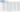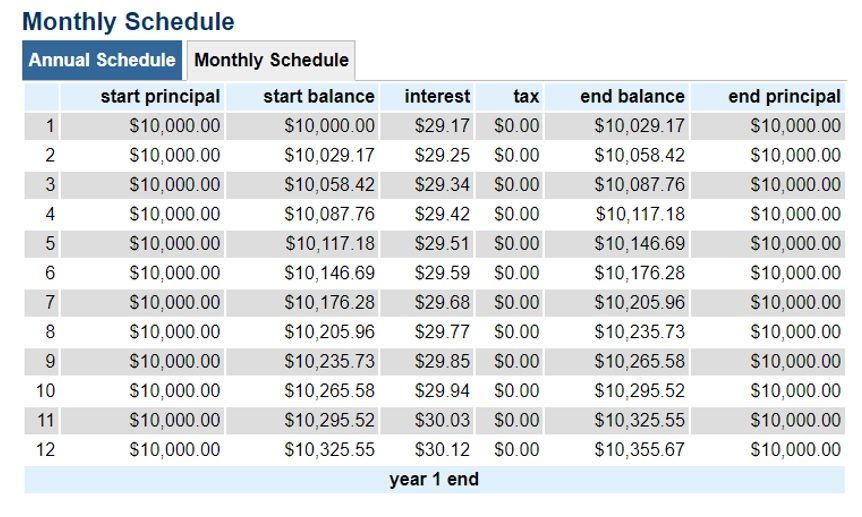Every month 3.5% of interest is applied to both the principal investment and any interest earned. Over time, this leads to exponential growth. So instead of earning 3.5% on \$10,000, investors will be earning 3.5% on \$10,029.17 after the first month, and so on.

## Earning Compound Interest on Crypto With APR

Investors who deposit crypto to APR-based earnings accounts can still earn compound interest. An earning product that quotes an APR will often still compound automatically – it just isn’t reflected in the interest rate. Investors may do it manually when interest doesn’t automatically compound. The potential compound frequency will depend on the lock-in periods for that particular earning product.

For example, interest and savings account for Bitcoin may mature every month. Until those 30 days are up, investors cannot touch their balance or withdraw their funds. This means the best possible compound frequency for those rates would be monthly (or 12 times per year).

Let’s say they deposited \$300 and earned another \$15 in that month. In this scenario, investors would need to re-invest both the \$300 and the \$15 yield into the next month’s earning period to receive compound interest. APY-based crypto earning accounts will generally complete this process automatically.Did you know?

The internet is full of interest calculators that can help investors figure out the most optimal strategy for earning on their cryptocurrency. Examples include the Interest Calculator and The Calculator Site.

## How To Calculate Interest with APY and APR?

Most crypto-earning products will show customers their rates, so no math has to be performed. Some even come equipped with in-built calculators to make the process easier. However, what if a customer wants to convert their APR to APY? Or, what if they only provide a flat interest rate? APY and APR have fairly simple formulas that investors can use to figure out exact rates.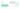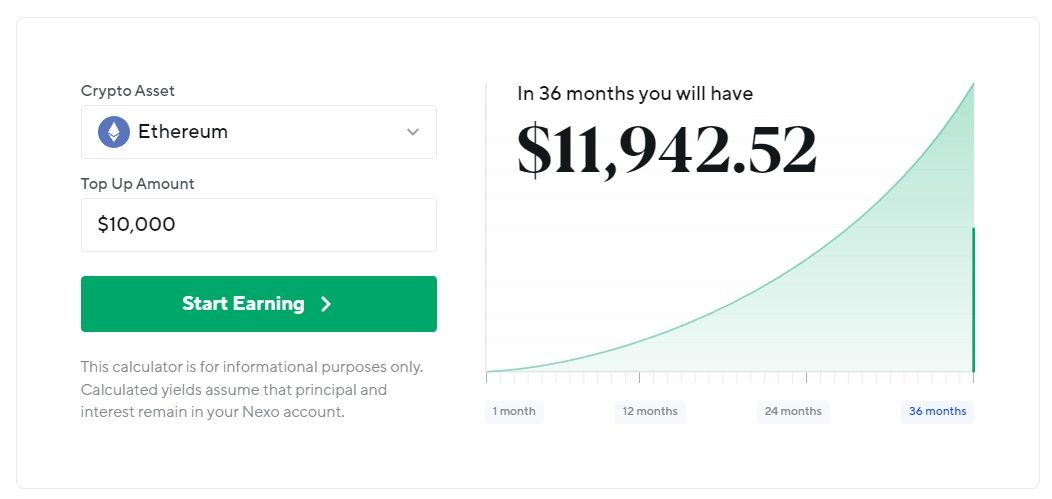Nexo interest calculator for earning on \$10,000 worth of Ethereum.

### Formula For Calculating APR

The formula for APR is as follows:

APR = Periodic rate x Number of periods in a year

This calculation is easy to work out. If an earning product offered an interest rate of 0.5% every month, its APR would be a flat 6%. Investors can then adjust this formula if they wish to determine their potential earnings from an APR.

Potential yield = Principal Investment x APR x Years/100

If an investor locked up 500 BTC at 10% APR for 5 years, the calculation would look like this:

500BTC x 10 x 5/100 = 250 BTC earned in interest

### Formula For Calculating APY

Calculating an APY is a little bit more complex than calculating an APR. It is still relatively straightforward and can be practical knowledge for serious DeFi users that wish to leverage yield farming strategies. The formula to calculate APR is:

APY = (1 + Interest rate / Number of periods in a year)^ Number of periods in a year – 1

First, the periodic rate must be converted from a percentage to a decimal. Divide the percentage by 100 to achieve this. A 6% annual rate that is compounded monthly would look like:

APY = (1 + 0.06 / 12)^12 - 1

The result of which is:

APY = 0.06167, or 6.167%

## How To Convert APR to APY In Crypto?

Not every earning product that lists an APR won’t compound. It just means the displayed rate doesn’t account for any compounding interest. Additionally, some earning tools won’t have a lock-in period, so the interest paid can be compounded as frequently as the investor wants. In this case, converting the listed APR to APY can be valuable. Earners can get a more accurate picture of their potential profits.

To convert APR into APY, substitute ‘Interest rate’ with the APR, and the ‘Number of periods’ with how frequently the yield will be re-invested. Remember to convert the APR to a decimal when using the formula:

APY = (1 + APR / Number of periods in a year)^ Number of periods in a year – 1

## Example of Converting APR To APY

Using Nexo as the example, which is one of the most popular lending platforms for crypto investors to build their wealth. The product accepts over 30 fiat currencies and supports earning/borrowing on 40+ digital currencies. Nexo offers up to 16% APR on Polygon (MATIC) and competitive rates on stablecoins (up to 12% APR). To learn what are stablecoins, read this article that explains how they work and their use cases.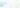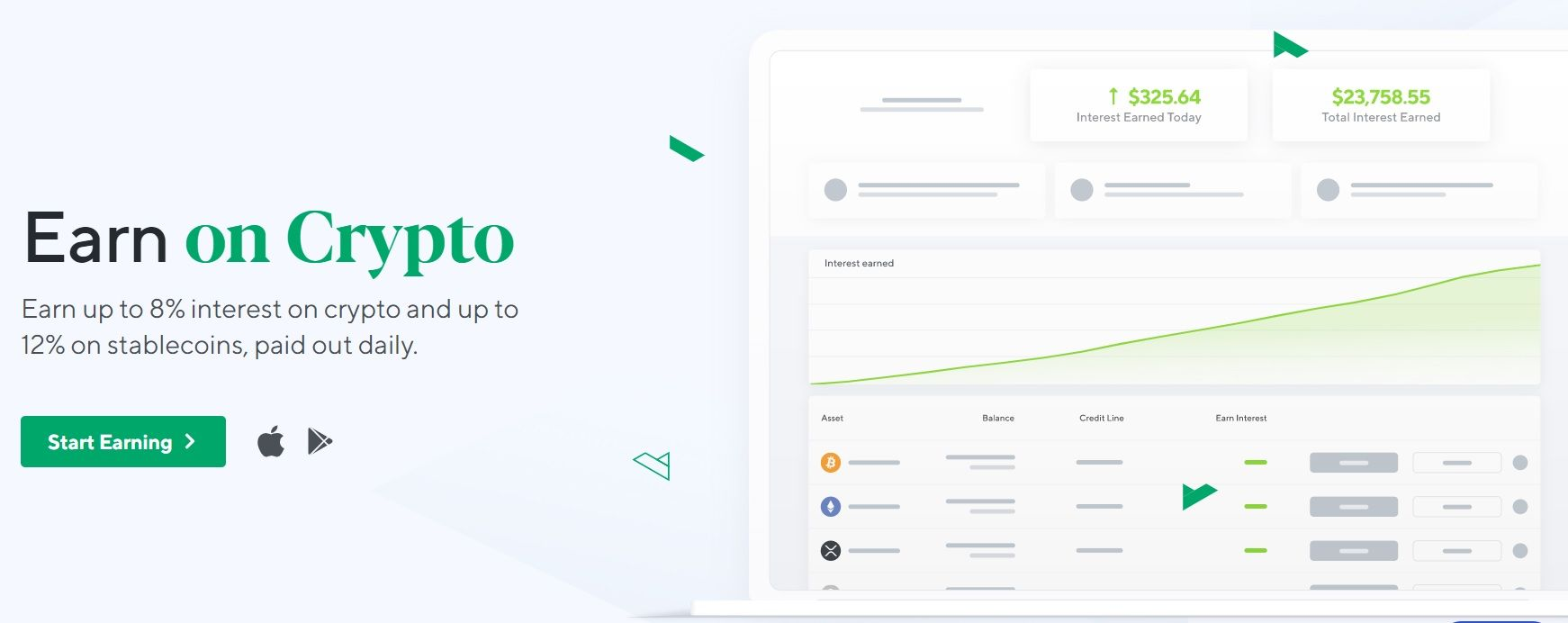The way Nexo structures its earning product is a great example of why understanding APR and APY is important in cryptocurrency. The platform offers daily compounding interest, whether you lock into a fixed one-three month contract, or a Flexi account (which can be accessed any time). However, Nexo’s quoted rates are always calculated as APR. Therefore, those earning on Nexo don’t get an accurate look at their APY.

The platform offers 5% APR on Bitcoin interest savings accounts when locked in for a month. It’s easy enough to figure out the earnings possibility by using their yield calculator which accounts for daily compounding. However, this is not as useful when comparing Nexo to another Bitcoin earning product to calculate annual interest using APY. So, investors must apply the formula to Nexo’s rates. The only information required is:

• APR – 5% (0.05)
• Compounding frequency – daily, denoted as 365 in the formula

The equation would therefore look like this:

APY = (1 + 0.05 / 365)^ 365 – 1

APY = 0.05126

Then multiply the answer by 100 to see the APY reflected as a percentage.

APY = 5.126%

Those who don’t want to do the math can use an online APR to APY converter instead.

## Risks of APY and APR

Interest rates in a vacuum don’t have any risks – they are just calculations of potential earnings. However, it is worthwhile to be wary of earning products with APYs that seem too good to be true. A frequent scam in the decentralized finance sector is a ‘rug pull’, or liquidity theft.

A malicious actor will release a new coin in a token pair with an unsustainably high APY (often over 500% APY). This may lure in new customers looking to maximize their earning potential. After a while, the scammer may remove the ability to withdraw tokens from the liquidity pool. They will then make off with all of the ‘valuable’ tokens in the pair, and eventually, the new coin’s value will drop to zero.

Annual percentage yield is a very useful way of understanding how much a lender can profit from digital currencies like Bitcoin. It accounts for compounding frequency and is therefore a more accurate depiction of yield than simple interest rates or APR.

APY refers to a compounding interest rate over an entire year. Different earning platforms will have various rules regarding the rate. Some APYs will be fixed for that entire year, whereas others are variable.

APY and APR in crypto are consistently higher than other financial products like savings accounts or term deposits. This is largely because cryptocurrencies are much riskier assets than fiat currency. It is also because there is more demand than supply for borrowing and lending crypto assets.

The rate of APY can vary dramatically depending on a lot of factors. DeFi products such as yield farming platforms such as Beefy.Finance is riskier and can produce rates of over 100%. Conversely, ‘safer’ earning methods like savings accounts average between 4–8%.

Yes, annual percentage yield in crypto refers to a yearly interest rate including compounding periods. On the other hand, APR does not include compounding frequency in its formula.

## Conclusion

Interest rates worldwide have hit record lows, which in turn has led to inflation of fiat currencies. The financial sector has been looking for a way to minimize the economic loss, culminating in the crypto earning boom in 2021. Earning on crypto through decentralized finance (DeFi) products offer greater interest rates than traditional financial tools. It presents a compelling opportunity for investors to put their digital currencies to work.

When embarking on a crypto yield journey, it is important to understand the difference between APR and APY. This knowledge is vital for comparing crypto interest earning platforms and figuring out profit margins. Though the amount of math involved in calculating interest seems intimidating, it is actually relatively simple. For those that wish to avoid math altogether, there are many tools out there that can automatically calculate interest in APR or APY, and interest earned over a specific period.

## You might also be interested inKevin Groves

Kevin started in the cryptocurrency space in 2016 and began investing in Bitcoin before exclusively trading digital currencies on various brokers, exchanges and trading platforms. He started HedgewithCrypto to publish informative guides about Bitcoin and share his experiences with using a variety of crypto exchanges around the world.